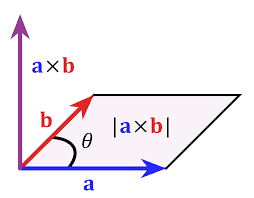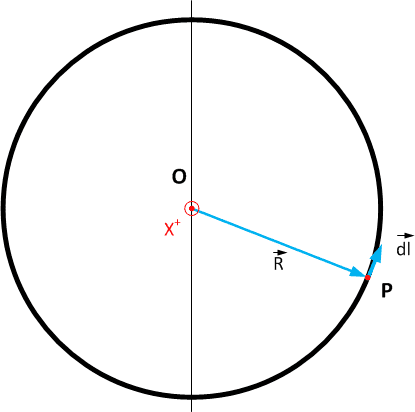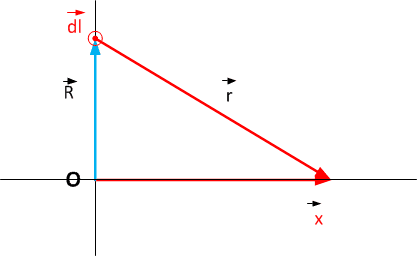# Magnetic field strength for circular loop

• Sho Kano

#### Sho Kano

When deriving the magnetic field strength due to a circular loop at some distance away from it's center (using Biot-Savart's law), why is the angle between ds and r 90 degrees?

This is a youtube video with the derivation, see 5:55

##\vec {dl}## is in the ##yz## plane. As drawn it is in the negative ##y## direction, so that ##\vec {dl} \perp \vec r## for the ##r## in the ##xz## plane. From Pythagoras you have ##R^2 + x^2 = r^2##. All ##\vec r## have the same length (from symmetry - rotation around the x-axis) - so this ##R^2 + x^2 = r^2## is true for all ##\vec r## -- so all these are rectangular triangles

The 90 degrees comes from the cross product in Biot-Savart's law.@Hesch: I would say the 90 degrees is used to simplify ##\vec a\times\vec b## to ##|\vec a||\vec b|## in BS, not that it comes from BS.

##\vec {dl}## is in the ##yz## plane. As drawn it is in the negative ##y## direction, so that ##\vec {dl} \perp \vec r## for the ##r## in the ##xz## plane. From Pythagoras you have ##R^2 + x^2 = r^2##. All ##\vec r## have the same length (from symmetry - rotation around the x-axis) - so this ##R^2 + x^2 = r^2## is true for all ##\vec r## -- so all these are rectangular triangles
r is not pointing straight out of the yz plane, so why is it perpendicular?

The ##\vec r## as drawn in the picture is in the xz plane. The ##\vec {dl}## as drawn is perpendicular to the xz plane: it is in the negative y direction. So that ##\vec {dl}## is perpendicular to that ##\vec r##.
For all other ##\vec {dl}## (in the yz plane) you can see they are ##\perp## 'their' ##\vec R## and also ##\perp## to the x-axis, so ##\perp## the plane in which their ##\vec R##, the x-axis and also ##\vec r##.

Bringing in Pythagoras was unnecessary - a mistake on my part.

@Hesch: I would say the 90 degrees is used to simplify ⃗a×⃗b\vec a\times\vec b to |⃗a||⃗b||\vec a||\vec b| in BS, not that it comes from BS
Sorry, maybe it's a danish term.
Try to write something in danish to me. Maybe I will comment it.The ##\vec r## as drawn in the picture is in the xz plane. The ##\vec {dl}## as drawn is perpendicular to the xz plane: it is in the negative y direction. So that ##\vec {dl}## is perpendicular to that ##\vec r##.
For all other ##\vec {dl}## (in the yz plane) you can see they are ##\perp## 'their' ##\vec R## and also ##\perp## to the x-axis, so ##\perp## the plane in which their ##\vec R##, the x-axis and also ##\vec r##.

Bringing in Pythagoras was unnecessary - a mistake on my part.
So just because yz is perpendicular to xz? There is no way dl is perpendicular to r because r extends from the yz plane down to xy, making an angle that is not 90 degrees.

No, not just because yz ##\perp## xz. Because ##\hat y \perp## xz for the situation as drwn in the video. In general:

Point P goes around the ring. At all such P $$\vec {dl}\perp \vec R \quad \& \quad \hat x \perp \vec {dl} \quad \Rightarrow \quad \vec {dl}\perp (\vec x - \vec R) = \vec r$$Hesch: jeg forstar lidt Dansk

#### Attachments

No, not just because yz ##\perp## xz. Because ##\hat y \perp## xz for the situation as drwn in the video. In general:

Point P goes around the ring. At all such P $$\vec {dl}\perp \vec R \quad \& \quad \hat x \perp \vec {dl} \quad \Rightarrow \quad \vec {dl}\perp (\vec x - \vec R) = \vec r$$

View attachment 100340

Hesch: jeg forstar lidt Dansk
I lost you at dl perpendicular to x - R, why did you do that? And why is it equal to r?

dl is perpendicular to ##\vec R##
dl is perpendicular to ##\hat x## so dl is perpendicular to ##\vec x##
that means dl is perpendicular to the plane in which both ##\vec R## and ##\vec x## lie.
That plane is described by ##a\vec x + b \vec R##.
The vector ##\vec r## is ##\vec R -\vec x## so it is in that plane.
Why is ##\vec r=\vec x -\vec R## ?
i don't know how to say that. Perhaps a picture helps ?#### Attachments

dl is perpendicular to ##\vec R##
dl is perpendicular to ##\hat x## so dl is perpendicular to ##\vec x##
that means dl is perpendicular to the plane in which both ##\vec R## and ##\vec x## lie.
That plane is described by ##a\vec x + b \vec R##.
The vector ##\vec r## is ##\vec R -\vec x## so it is in that plane.
Why is ##\vec r=\vec x -\vec R## ?
i don't know how to say that. Perhaps a picture helps ?

View attachment 100362
Okay, I see where x - R comes from.

So this is how I'm comprehending it:
dl is pointing outwards (imagine a wire pointing out), so any r from its surface is perpendicular to it.
Before, I was confusing dl with the z vector. The angle that r makes with z will not be perpendicular.

I think the reason is that they look for field only along the ##z## axis. I guess there the integral can be solved analytically, while the field of the current-conducting circular loop at an arbitrary position leads to elliptic integrals.